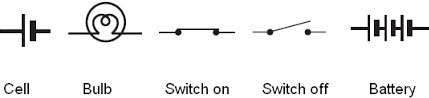Wednesday , October 5 2022# NCERT 7th Class (CBSE) Science: Electric Current and its effects

#### Question: What is an electric circuit?

Answer: An electric Circuit is a continuous conducting path for electric current.

#### Question: What is the relationship between potential difference and direction of flow of current in an electric circuit?

Answer: If current flows from point A to point B in a wire, we say that A is at a higher potential that B or that there is a potential difference between A and B.

#### Question: Does every conductor heat up when an electric current is passed through it? What does the amount of heat depend on? Name the conductor normally used in heating appliances.

Answer: No every conductor does not heat up when an electric current is passed through it. The amount of heat depends upon the resistance offered by the conductor and the length and thickness of the conductor.

#### Question: A domestic circuit develops a short circuit. What will be the effect on a fuse wire connected in the circuit?

Answer: If domestic circuit develops a short circuit then the fuse wire connected in the circuit will melt.

#### Question: What is a miniature circuit breaker?

Answer: Miniature circuit breaker (MCB) is used instead of or in addition to fuses, in the household electric circuits. It is a switch that automatically stops the current in a circuit. Nichrome and tungsten are used in heating appliances if the current in it exceeds the specified maximum limit.

#### Question: Draw the symbol used in a circuit for – a battery of four cells, a switch (ON and OFF), a lamp (bulb).## Sources of Energy: 10th Science Chapter 14

Class: 10th Class Subject: Science Chapter: Chapter 14: Sources of Energy Quiz: – Questions MCQs: …

1.2.3.